Math Modeling : Computational Methods

Example Questions

Example Question #3 : Optimization Models

Using Newton's Method as the computational method, optimize the follow problem.

A cow that weighs 175 pounds gains 3 pounds per day, and it costs 30 cents per day to keep that cow. The market price for cows is 75 cents per pound, but the price is falling one cent per day. When should the cow be sold to make the maximum profit?

It is recommended to wait 6 or 7 days to sell the cow. This should result in a net profit of $130. It is recommended to wait 7 or 8 days to sell the cow. This should result in a net profit of$131.90.

It is recommended to wait 9 or 10 days to sell the cow. This should result in a net profit of $132. It is recommended to wait 2 or 5 days to sell the cow. This should result in a net profit of$131.90.

It is recommended to wait 8 or 9 days to sell the cow. This should result in a net profit of $132.90. Correct answer: It is recommended to wait 7 or 8 days to sell the cow. This should result in a net profit of$131.90.

Explanation:

Assume that the growth rate of the cow is proportional to its weight.

In mathematical terms: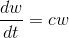Looking at the known information,

Variables:Therefore,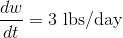when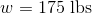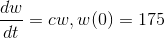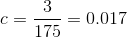Thus the differential equation to solve for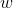is as follows.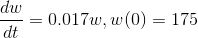Solving by separation of variables results in the following equation.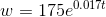From here, formulate the model with the new weight equation.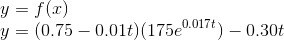Recall that the goal is to optimize the question over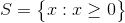.

Use a graphing calculator or computer technology to graph the function.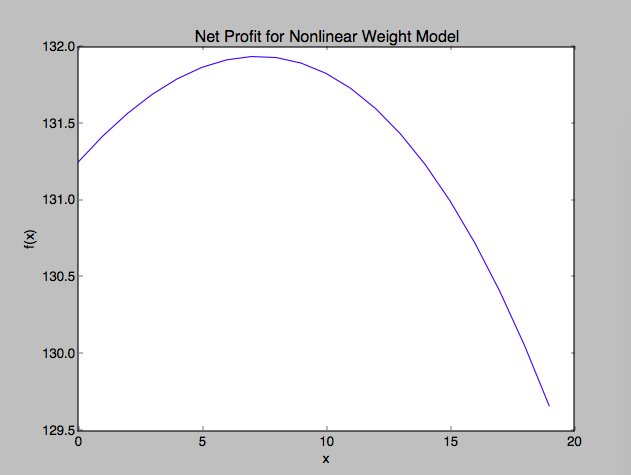Observing the graph it appears the maximum profit for the cow occurs around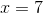and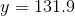.

Example Question #2 : Linear Programming

A farm has 750 acres of land available for planting crops. The possible crops are soy, corn, and oats. There is 1,200 acre-ft of water for irrigation and the crew will be able to work of the farm 575 hours per week.

Find the amount of each crop that should be planted to maximize the profit.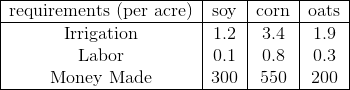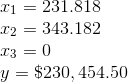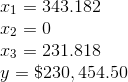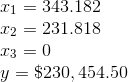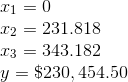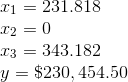Explanation:

The objective of this particular problem is to maximize the profit.

Maximize the function: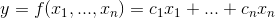over the region defined by the constraints.

First identify the variables and assumptions of the problem.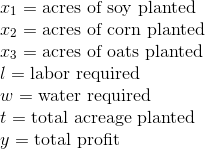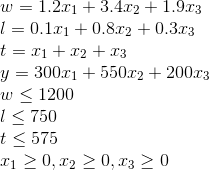Now using the constraints formulate the linear programming model.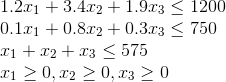From here use computer implementation to solve using the simplex method.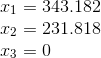Therefore, the optimal solution will occur when there are 343.182 acres of soy planted and 231.818 acres of corn planted.

The maximum profit at this solution will be, \$230,454.50.